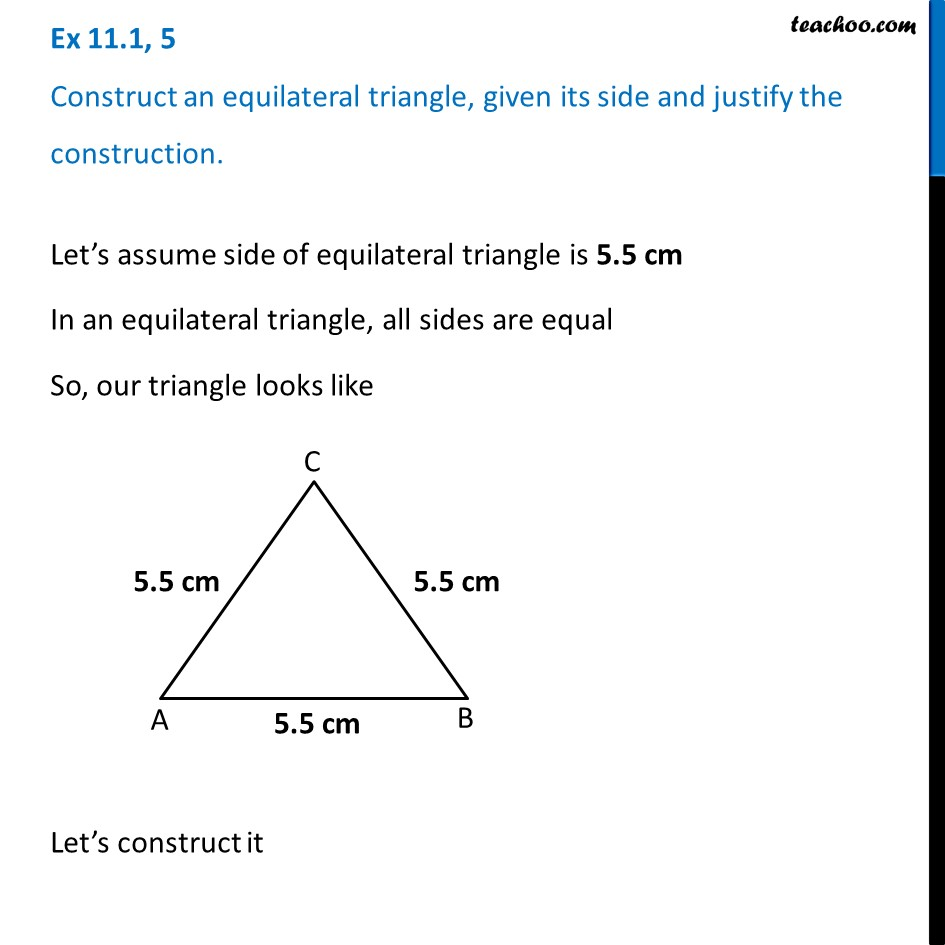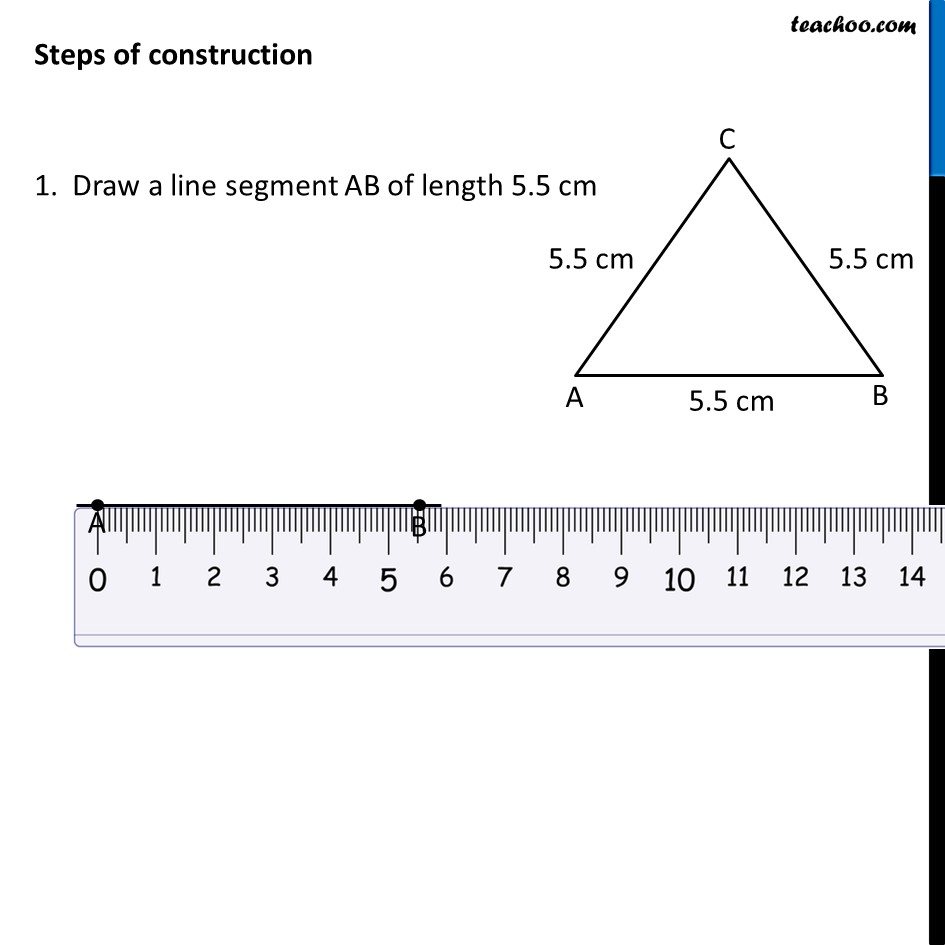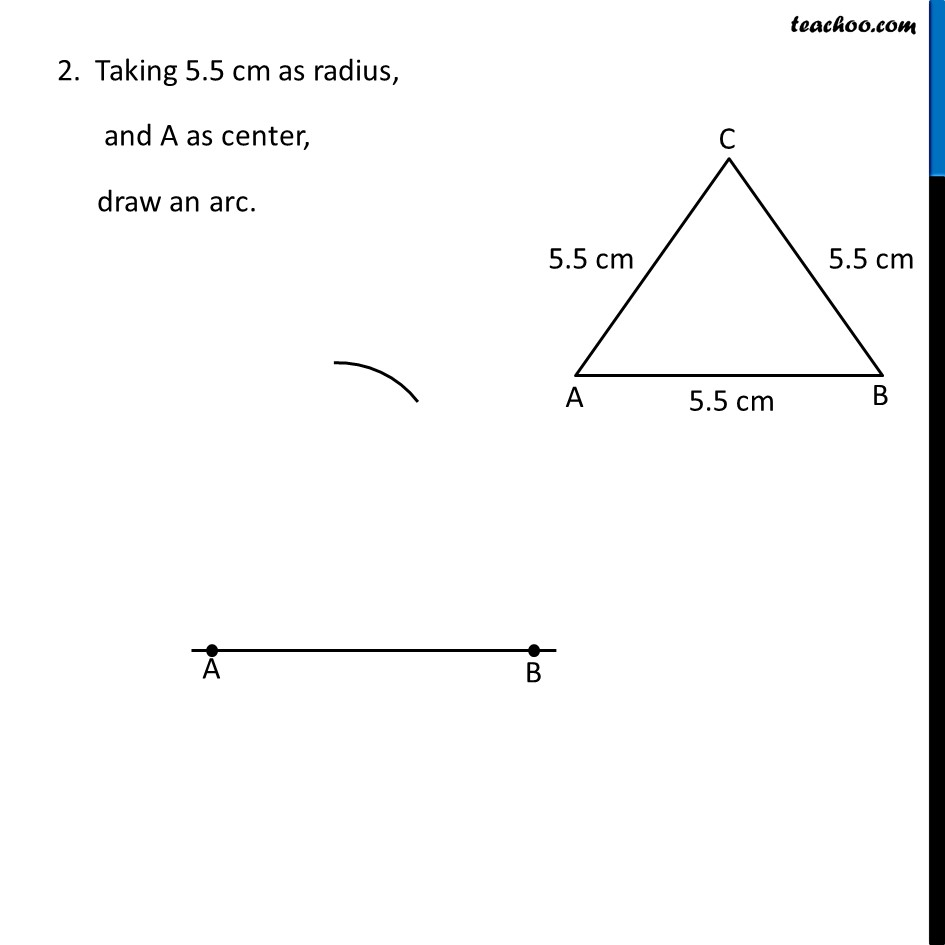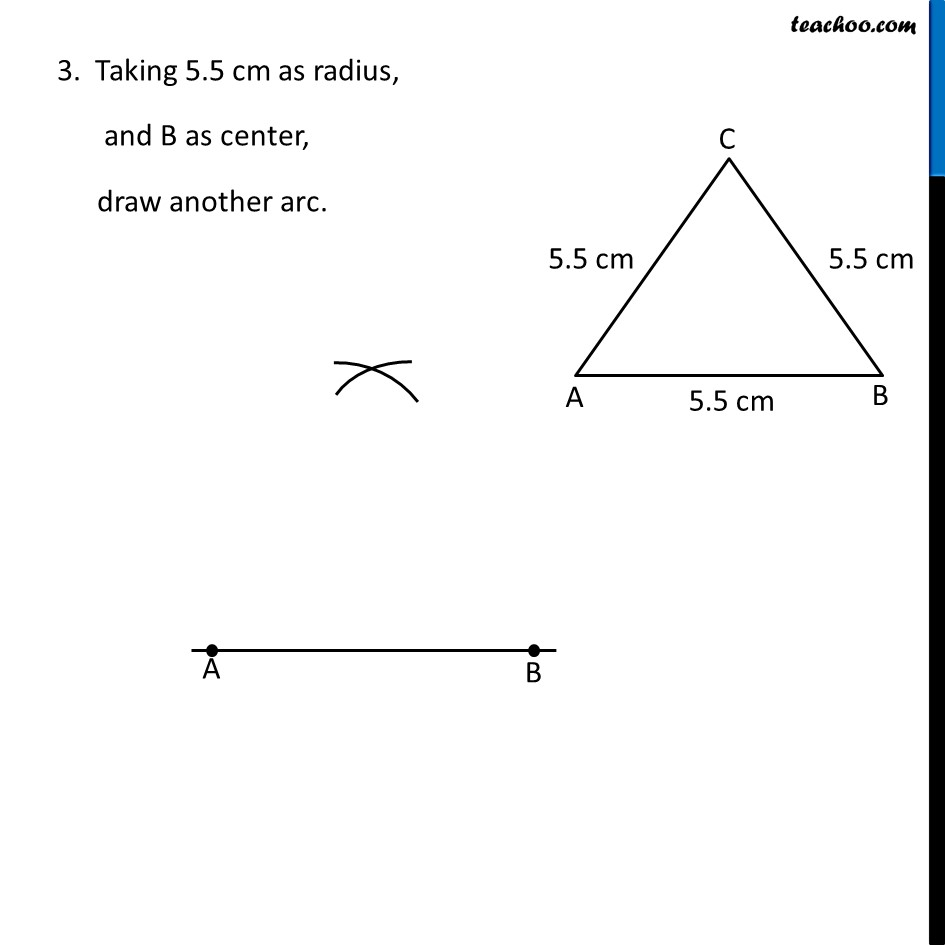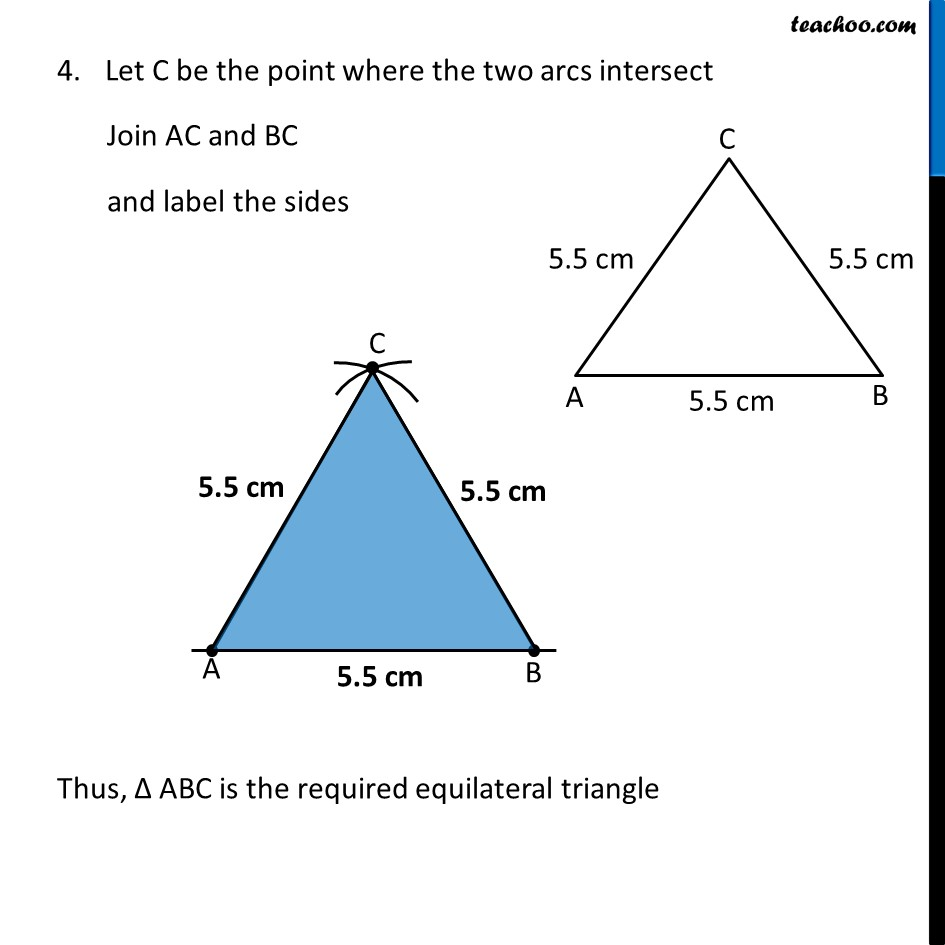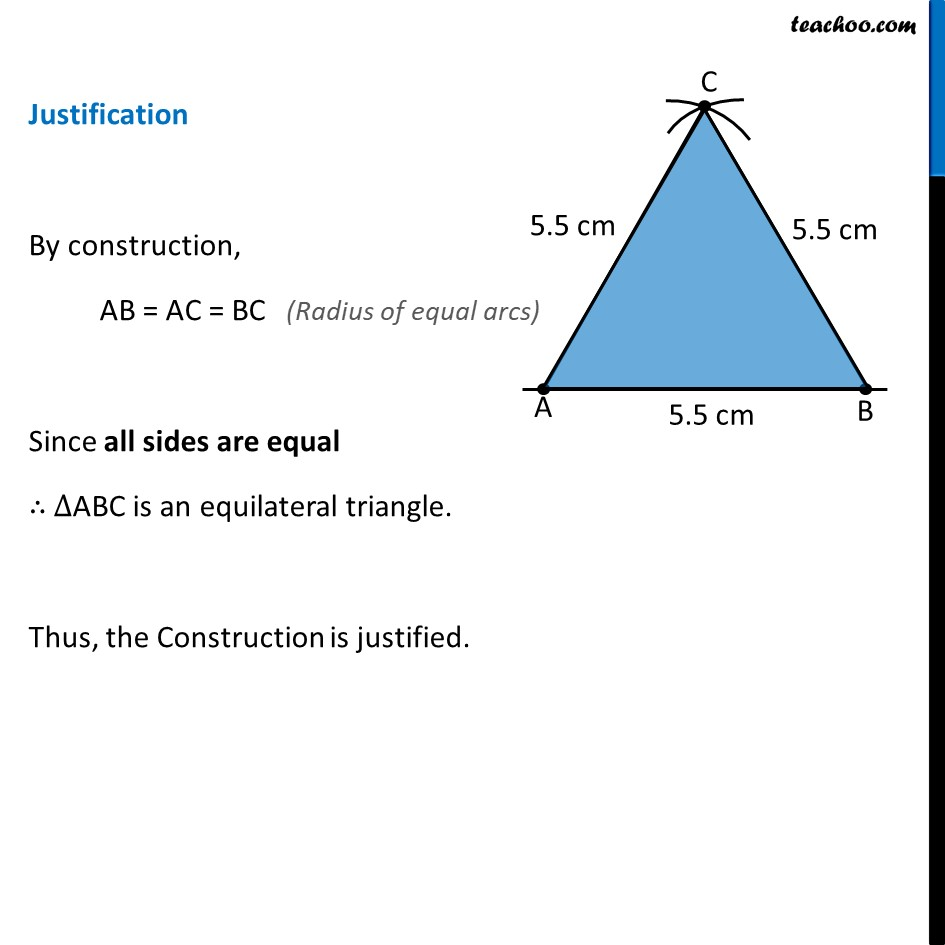1. Chapter 11 Class 9 Constructions
2. Concept wise
3. Constructing bisector of angle and perpendicular bisector

Transcript

Ex 11.1, 5 Construct an equilateral triangle, given its side and justify the construction.Let’s assume side of equilateral triangle is 5.5 cm In an equilateral triangle, all sides are equal So, our triangle looks like Let’s construct it Steps of construction 1. Draw a line segment AB of length 5.5 cm 2. Taking 5.5 cm as radius, and A as center, draw an arc. 3. Taking 5.5 cm as radius, and B as center, draw another arc. Let C be the point where the two arcs intersect Join AC and BC and label the sides Thus, Δ ABC is the required equilateral triangle Justification By construction, AB = AC = BC Since all sides are equal ∴ ∆ABC is an equilateral triangle. Thus, the Construction is justified. (Radius of equal arcs)

Constructing bisector of angle and perpendicular bisector

About the AuthorDavneet Singh
Davneet Singh is a graduate from Indian Institute of Technology, Kanpur. He has been teaching from the past 10 years. He provides courses for Maths and Science at Teachoo.